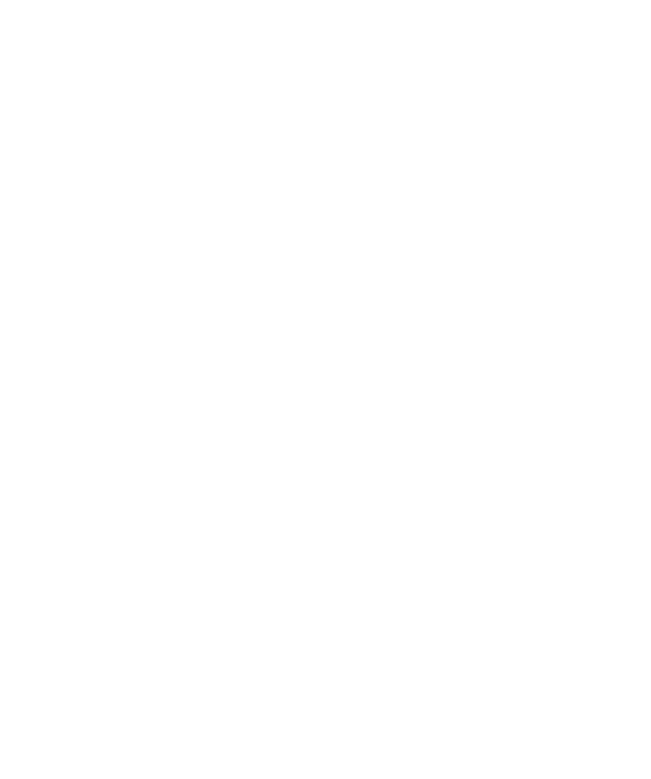Mathematical and Theoretical Physics

Program director: Igor Krichever

Also implemented in network form with Higher School of Economics (HSE), Moscow Institute of Physics and Technology (MIPT)
Courses implemented with partner institutes marked with *
Track "Mathematical physics"
Track "Theoretical physics"
Research seminar "Supersymmetric Gauge Theories and Integrable Systems"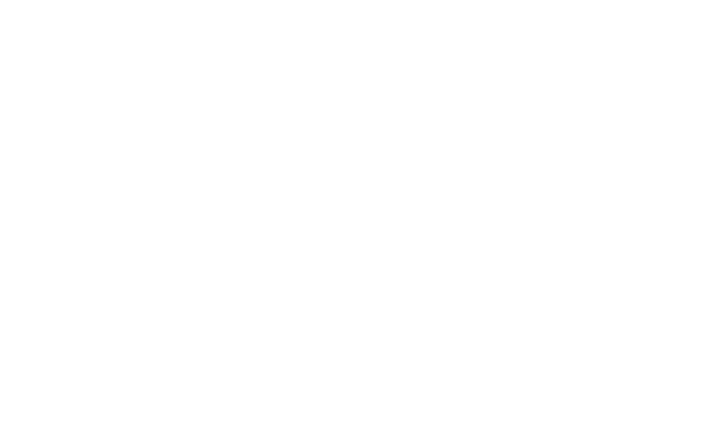Representations of Classical Groups and Related Topics*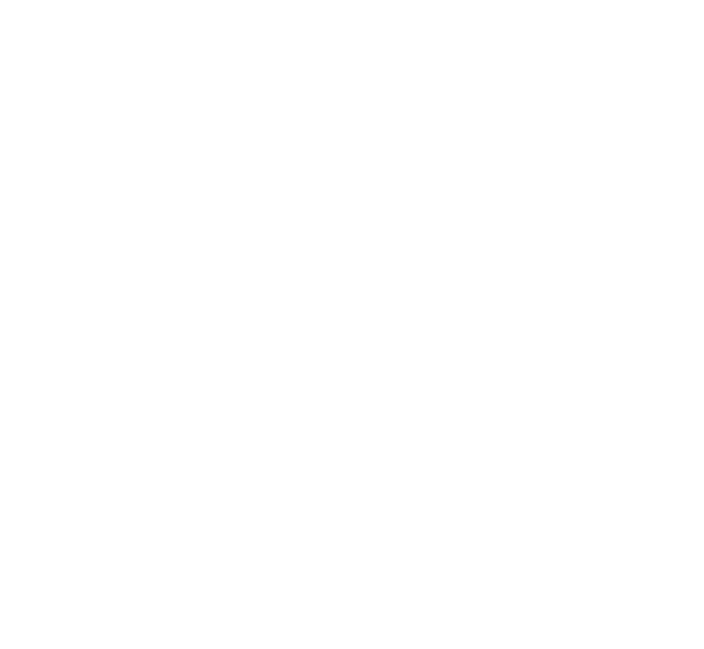Geometric Representation Theory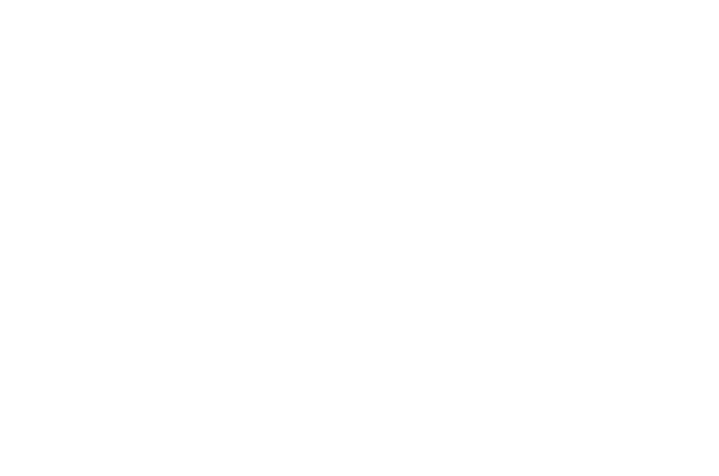Elliptic Operators in Topology of Manifolds*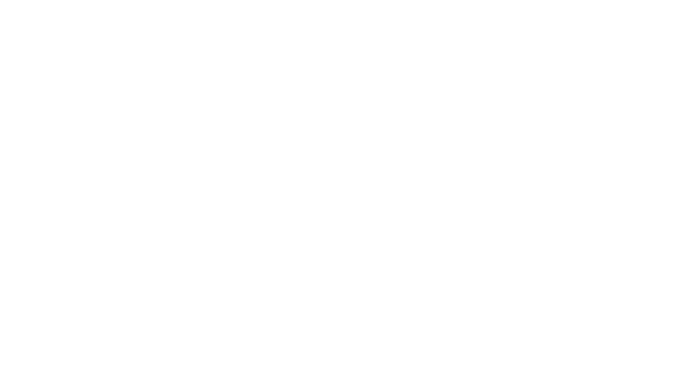Classical Integrable Systems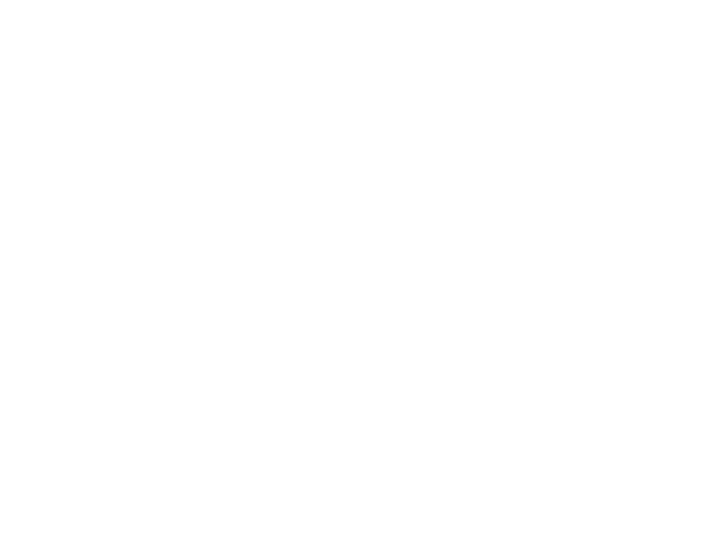Phase Transitions: Introduction to Statistical Physics and Percolation*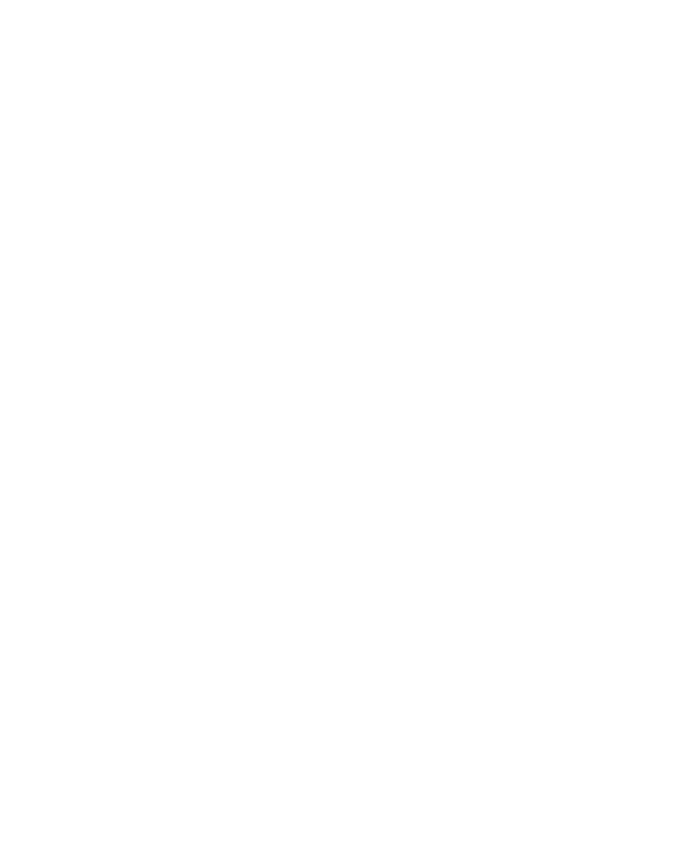Quantum Mechanics*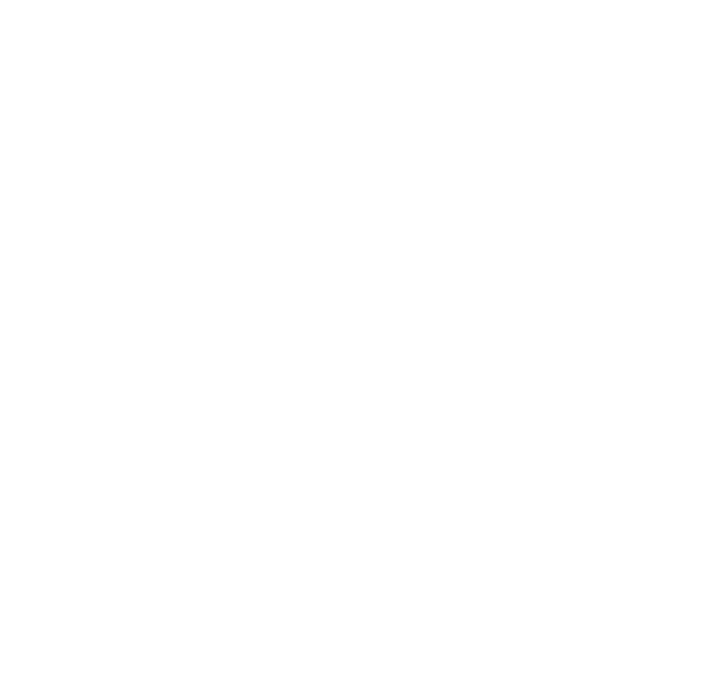Mathematical methods of science*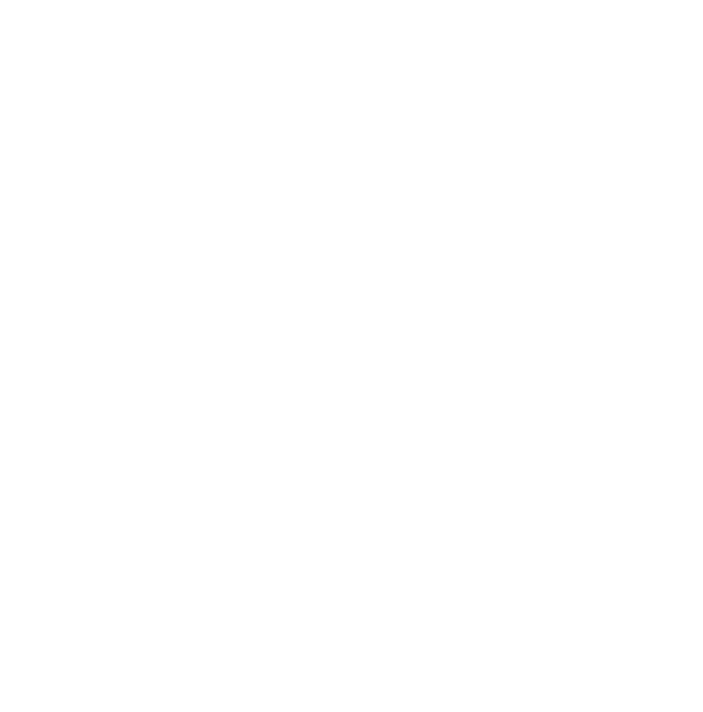Critical points of functions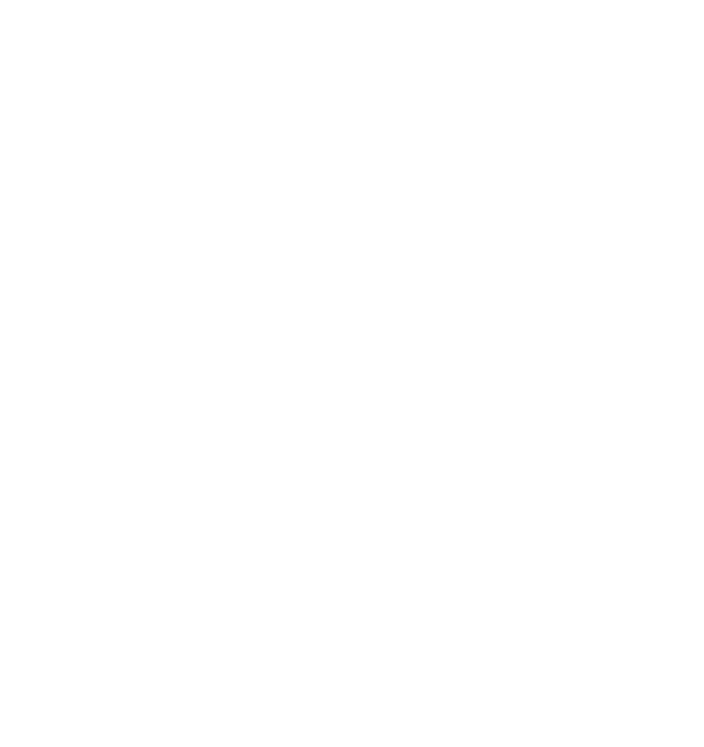Introduction to quantum groups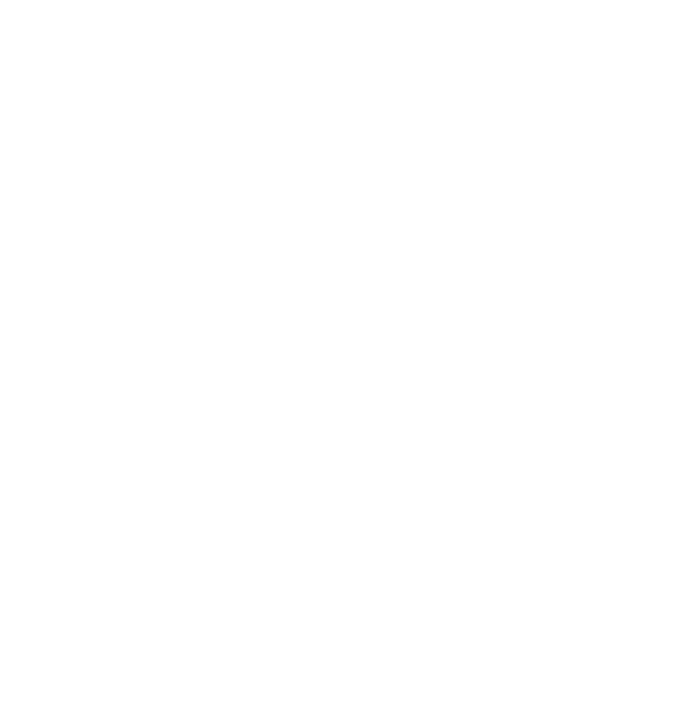Modern Dynamical Systems*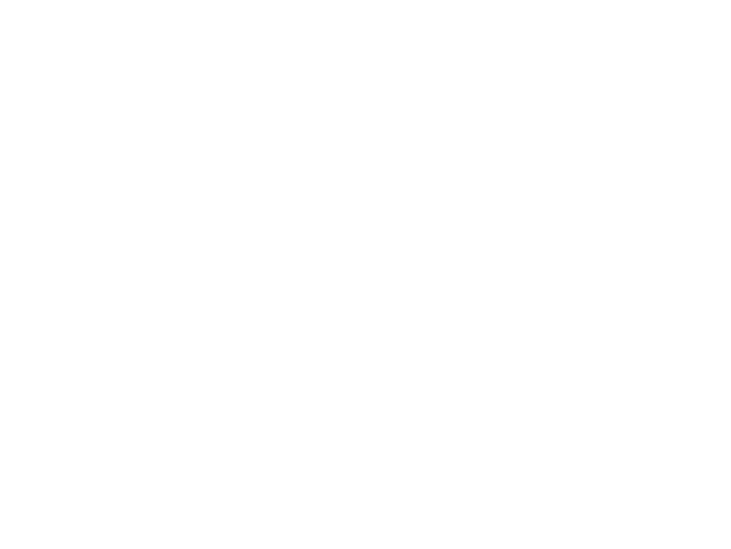Gauge Fields and Complex Geometry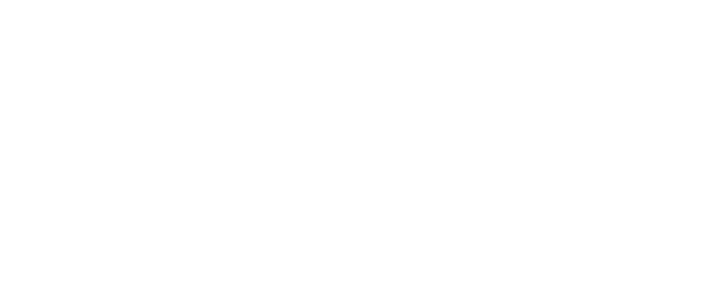Quantum Integrable Systems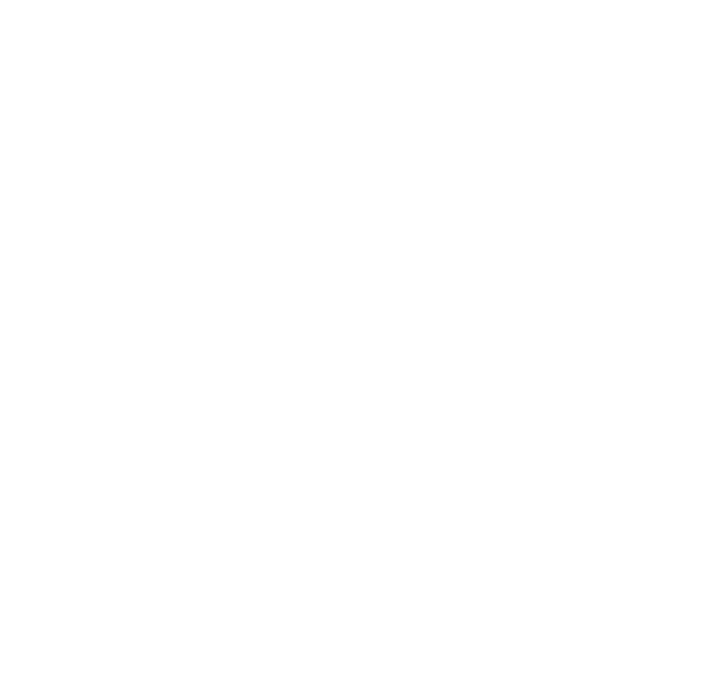Quiver Representations and Quiver Varieties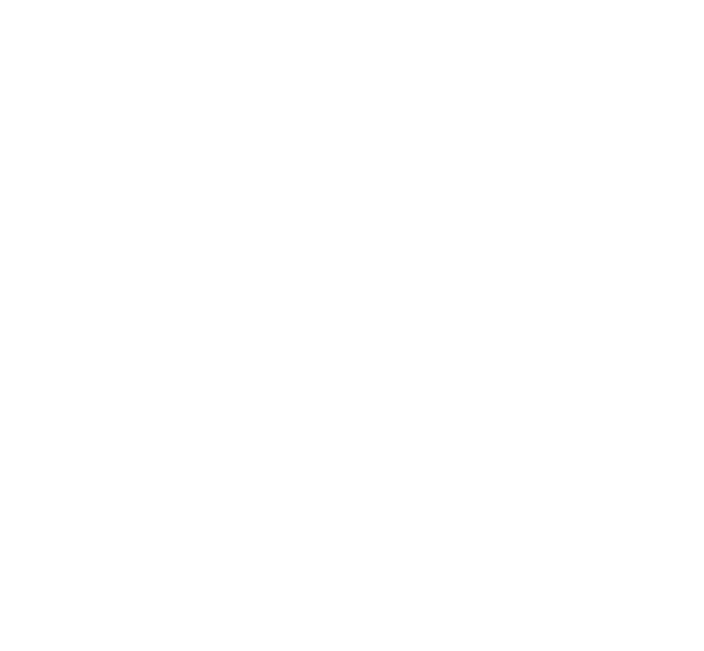Introduction to Quantum Theory*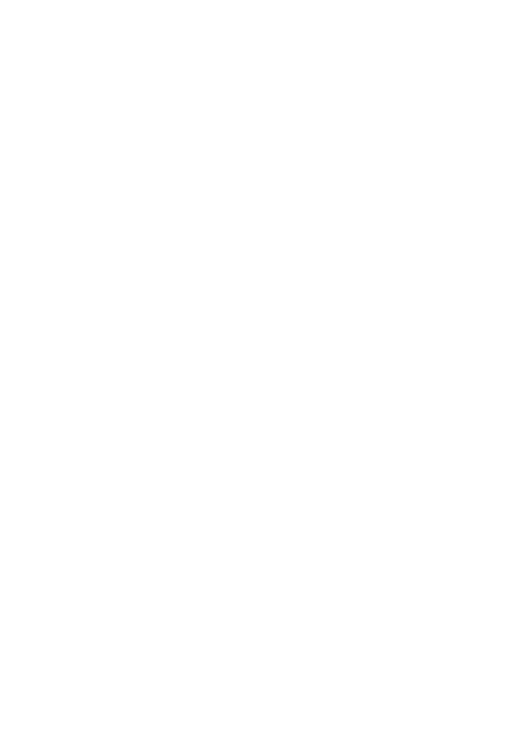Quantum Field Theory*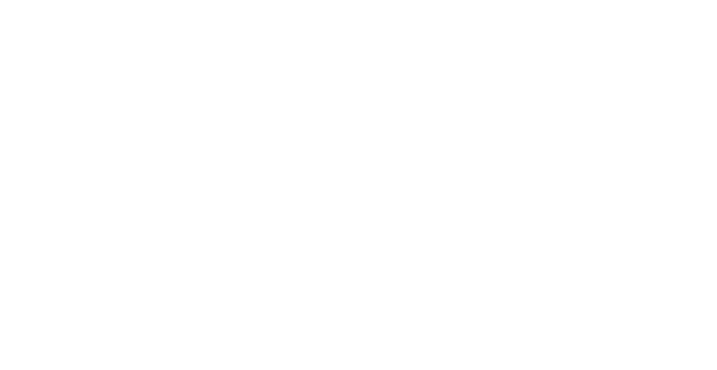Advanced Quantum Mechanics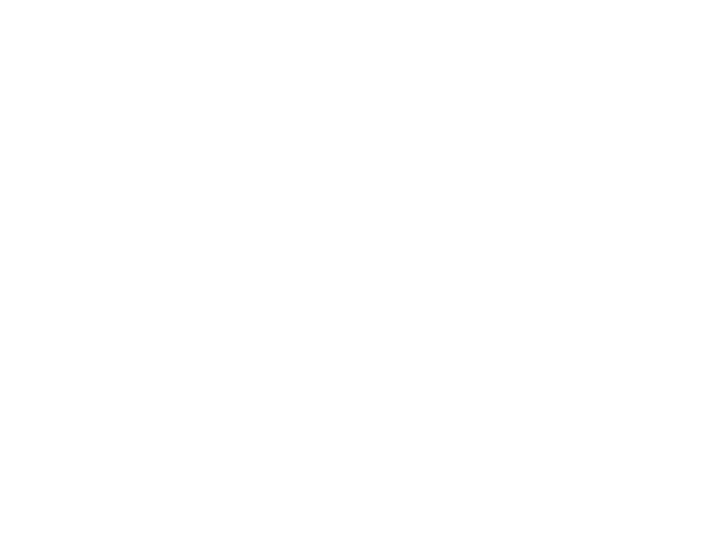Path Integrals and Physics of Open Quantum Systems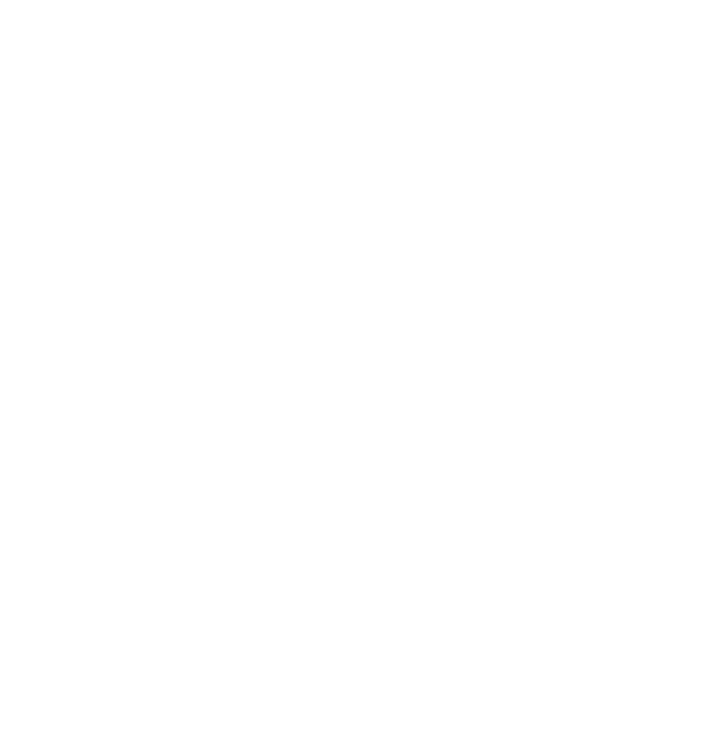Functional Methods in the Theory of Disordered Systems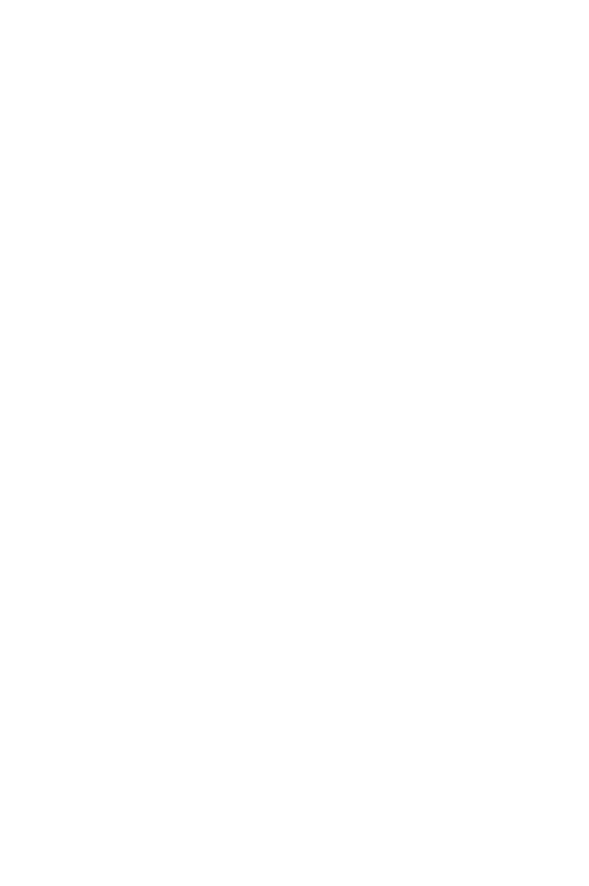Theory of Phase Transitions*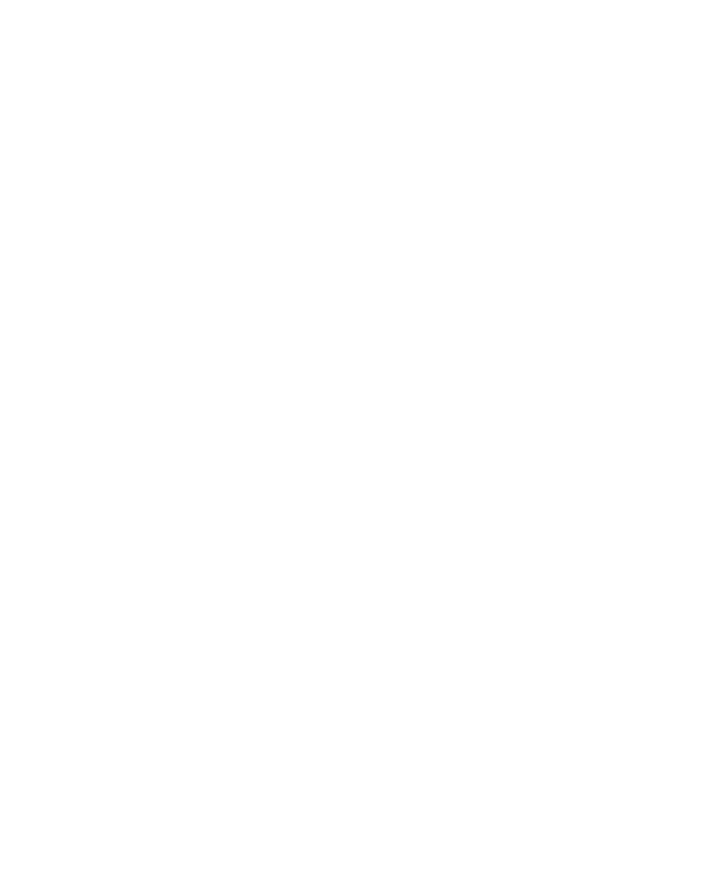Introduction to the Theory of Disordered Systems*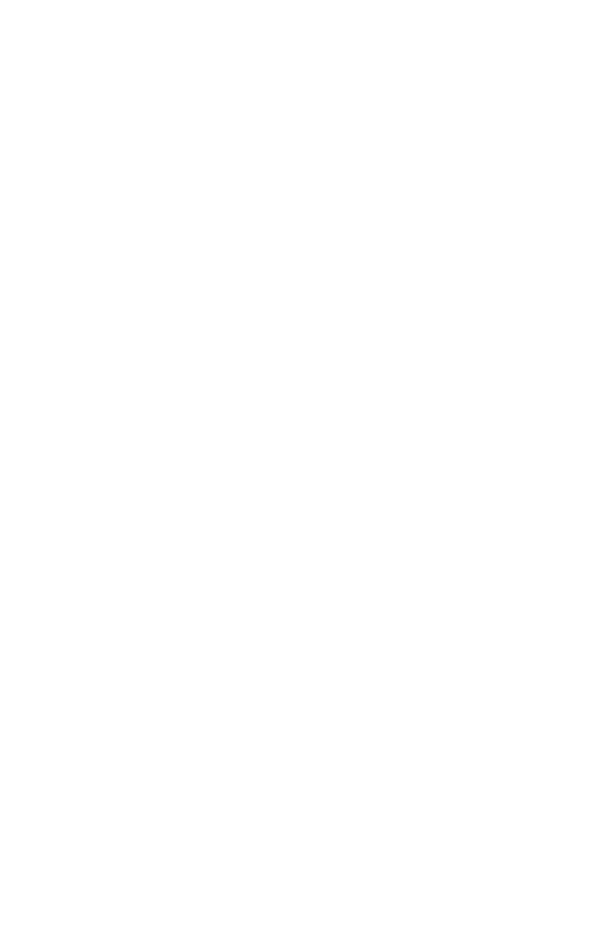Introduction to the Quantum Field Theory*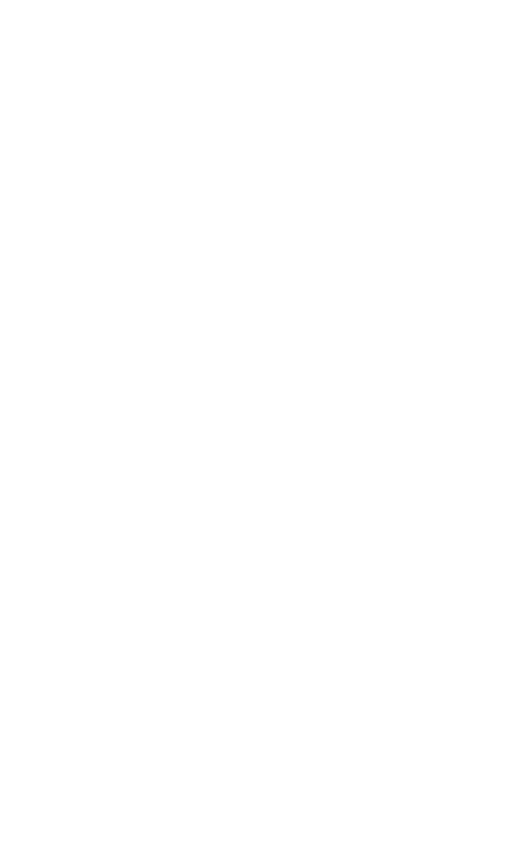Numerical Simulations of Quantum Many-Body Systems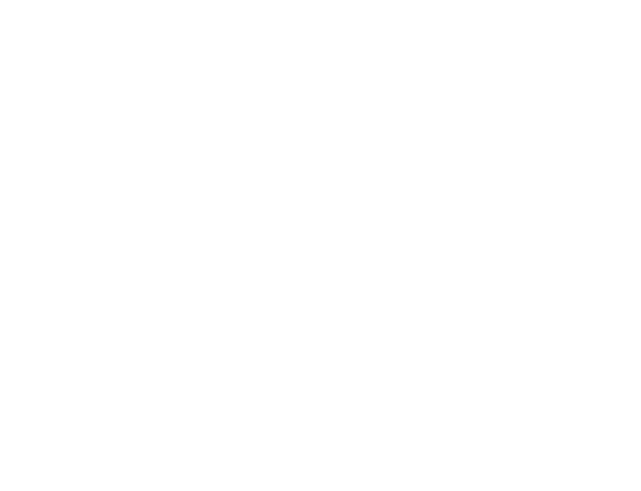Quantum Mesoscopics. Quantum Hall effect*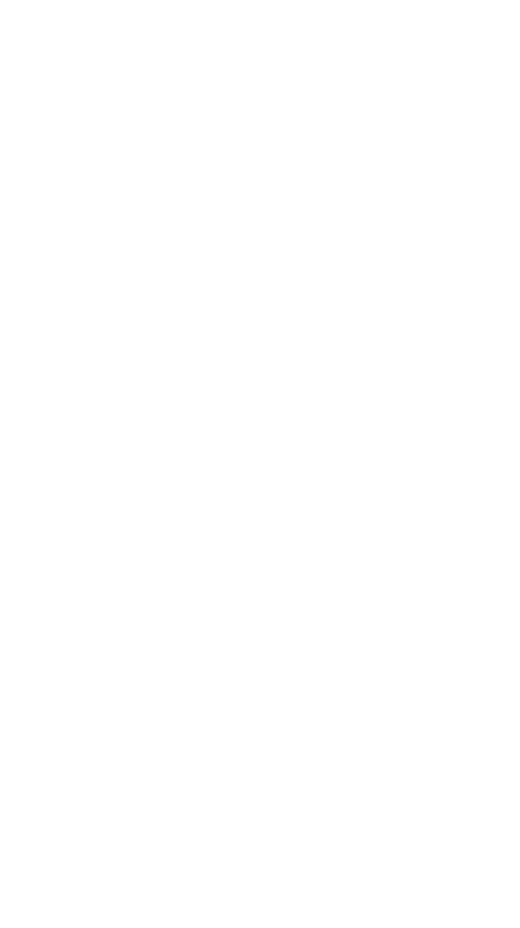One-Dimensional Quantum Systems*